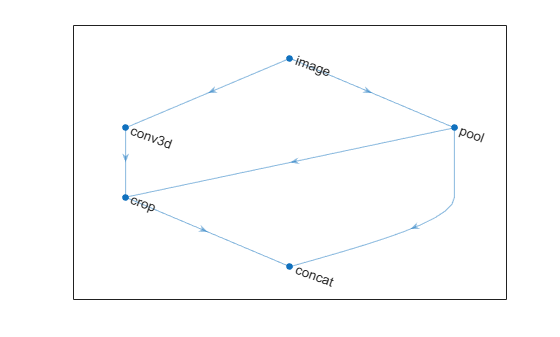Documentation

# crop3dLayer

3-D crop layer

## Description

A 3-D crop layer crops a 3-D volume to the size of the input feature map.

Specify the number of inputs to the layer when you create it. The inputs to the layer have the names `'in'` and `'ref'`. Use the input names when connecting or disconnecting the layer by using `connectLayers` or `disconnectLayers`. All inputs to a 3-D crop layer must have the same number of dimensions.

## Creation

### Syntax

``layer = crop3dLayer``
``layer = crop3dLayer([X Y Z])``
``layer = crop3dLayer(___,'Name',Name)``

### Description

````layer = crop3dLayer` creates a 3-D crop layer that crops an input feature map from the center of the feature map. The size of the cropped region is equal to the size of a second reference input feature map.```
````layer = crop3dLayer([X Y Z])` also sets the cropLocation property with the (X,Y,Z) coordinate of the crop window. X is the coordinate in the horizontal direction, Y is the coordinate in the vertical direction, and Z is the coordinate in the depth direction.```

example

````layer = crop3dLayer(___,'Name',Name)` also sets the `Name` property. To create a network containing a 3-D crop layer, you must specify a layer name.```

## Properties

expand all

### Crop

Crop location, specified as `'centercrop'` or a three-element numeric vector representing the (x,y,z) coordinate of the crop window.

### Layer

Layer name, specified as a character vector or a string scalar. To include a layer in a layer graph, you must specify a nonempty unique layer name. If you train a series network with the layer and `Name` is set to `''`, then the software automatically assigns a name to the layer at training time.

Data Types: `char` | `string`

Number of inputs of the layer. This layer accepts two inputs.

Data Types: `double`

Input names of the layer, specified as `{'in','ref'}`. This layer accepts two inputs.

Data Types: `cell`

Number of outputs of the layer. This layer has a single output only.

Data Types: `double`

Output names of the layer. This layer has a single output only.

Data Types: `cell`

## Examples

collapse all

Create a 3-D crop layer and connect both of its inputs using a layerGraph object.

```layers = [ image3dInputLayer([32 32 32 3],'Name','image') convolution3dLayer(3,16,'Padding','same','Name','conv') crop3dLayer('Name','crop') concatenationLayer(4,2,'Name','concat') ]```
```layers = 4x1 Layer array with layers: 1 'image' 3-D Image Input 32x32x32x3 images with 'zerocenter' normalization 2 'conv' Convolution 16 3x3x3 convolutions with stride [1 1 1] and padding 'same' 3 'crop' Crop 3D center crop 4 'concat' Concatenation Concatenation of 2 inputs along dimension 4 ```

Create a layer graph. The first input of the 3-D crop layer is automatically connected to the output of the 3-D convolutional layer.

`lgraph = layerGraph(layers);`

Add a max pooling layer to the layer graph.

```maxPool = maxPooling3dLayer(2,'stride',2,'Name','pool'); lgraph = addLayers(lgraph,maxPool); lgraph = connectLayers(lgraph,'image','pool');```

Connect the second input of the crop layer to the output of the max pooling layer.

`lgraph = connectLayers(lgraph,'pool','crop/ref');`

Concatenate the crop layer output and the max pooling layer output.

`lgraph = connectLayers(lgraph,'pool','concat/in2');`

Display the layer graph.

`plot(lgraph)`